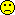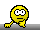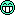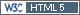ElMaestro
Hero

Denmark,
2009-05-20 20:35
(edited by ElMaestro on 2009-05-21 10:29)

Posting: # 3719
Views: 9,117

## Mean as intercept; model matrices [R for BE/BA]

Dear all,

I have two questions about R and BE and the model specification of Chow & Liu. In principle, I could ask these questions in the more dedicated R fora, however, since Bebac users are friendlier and sexier and probably are able to provide answers that relate directly to BE, I prefer to ask them here.

For illustration purposes I have invented some arbitrary data, in R syntax:
``` lnY  = c(10,11,12,13,14,15,10,11,12,13,14,15,16,17) Per  = as.factor(c(1,1,1,1,1,1,1,2,2,2,2,2,2,2)) Seq  = as.factor(c(1,1,1,1,2,2,2,1,1,1,1,2,2,2)) Trt  = as.factor(c(1,1,1,1,2,2,2,2,2,2,2,1,1,1)) Subj = as.factor(c(1,2,3,4,5,6,7,1,2,3,4,5,6,7)) Snot = lm(lnY~Trt+Subj+Seq+Per)``` ## seems to be standard syntax in this forum
...which corresponds to a 2,2,2-BE study with 7 subjects

1. Chow & Liu's model specification is
lnY = Mean + S(i,k) + P(j) + F(j,k) + C(j-1,k) + E(i,j,k)
The `lm` fit above is not exactly corresponding to this model, as `lm` will fit an intercept unless we request it specifically not to. The fitted intercept is not the mean. This makes no difference in terms of the anova/error term BUT it will have some consequence for the regressors. Luckily, when we calculate the 90% CI, we do not need the individual model constants for the two treatments, we actually only need the difference between the model coefficients for the two treatments [rule: ln(A)-ln(B)=ln(A/B)], and that difference is preserved regardless of the magnitude of the intercept. We can get the difference from `summary(Snot)`, where we read out how different Trt2 is from Trt1.
But let's assume we want to do the fit as Chow & Liu want us to. Which `lm` syntax works? I cannot do it with `lm` or `glm`. The only way I can do achieve the same is via the absolutely horrendous syntax:
``` Snot2 = lm(lnY-mean(lnY)~Trt+Subj+Seq+Per-1)``` or:
`lnY2  = lnY-mean(lnY)` and then `Snot2 = lm(lnY2~Trt+Subj+Seq+Per-1)`

The advantage of Snot2 is that we ask R to do a fit without the intercept (we have subtracted the intercept/mean on the left-hand side anyway). Now we can read out both treatment regressors directly from `summary(Snot2)`, not just the difference between them.
Is there a smarter, direct way of doing the fit the Chow&Liu way with mean as intercept?

2. In principle the overall model matrix for Snot above will have 14 rows (14 observations) and 14 columns (1 intercept+7 subjects+2 periods+2 treatments+2 sequences). Then there are redundancies so the 14×14 matrix will not be full rank, making it difficult to do matrix boogie (like inversion etc, which can be really handy when fitting) on it. Some statistical packages therefore prefer to reduce the model matrices to full rank. Now let's look at R's model matrix for Snot:
``` model.matrix(Snot) ```
On my computer, I get a matrix with 10 columns. Obviously, the column called "Seq2" can be constructed from the three columns "Subj5", "Subj6" and "Subj7". Thus the muddle matrix is not full rank. It has rank=9 [`qr(model.matrix(Snot))`].
So, why on earth will R reduce a model matrix into something that does not have full rank? What is the computational advantage of doing the muddle fit on this matrix?

Many thanks for any insight you can provide
EM.

--
Edit1: Formatted using BBCode. [Helmut]
Edit2: Originally got 15 when I added 1,7,2,2 and 2. Corrected above. Morale: Never trust any calculation done by ElMaestro.
Edit3: Welcome to the club! [Helmut]
yjlee168
SeniorKaohsiung, Taiwan,
2009-05-21 13:35

@ ElMaestro
Posting: # 3721
Views: 8,183

## Mean as intercept; model matrices

dear Elmaestro,

So far we still cannot figure out why the intercept is not the mean of lnY. However, you put Subj as one of regressors in your lm() functions. In Chow & Liu's model, the S(i,k) is the intersubject residuals of Subj i on Sequence k, not Subj itself. Could this be the reason that these two models might be different? What we use in bear with your example is like the following:
`Snot <- lm(lnY~Trt+Subj:Seq+Per+Seq)` for a 2x2x2 ABE. It works o.k..

» Snot = lm(lnY~Trt+Subj+Seq+Per) ## seems to be standard syntax in
» 1. Chow & Liu's model specification is
» lnY = Mean + S(i,k) + P(j) + F(j,k) + C(j-1,k) + E(i,j,k)

All the best,
---Yung-jin Lee
bear v2.8.3-3:- created by Hsin-ya Lee & Yung-jin Lee
Kaohsiung, Taiwan http://pkpd.kmu.edu.tw/bear
ElMaestro
Hero

Denmark,
2009-05-21 14:30

@ yjlee168
Posting: # 3722
Views: 8,148

## Mean as intercept; model matrices

Hi Bears
» `Snot <- lm(lnY~Trt+Subj:Seq+Per+Seq)` for a 2x2x2 ABE. It
» works o.k..

I guess you are right, but I think this is fishy. I could be wrong, but the colon as far as I understand from the manual is used for interactions?! Try and look at the model matrix with your syntax, where you will see columns filled with zeros. For example, with these data you will see a column called "Subj2:Seq2" which consists of all zeros, simply because Subj2 is assgined to Seq1 (and therefore the column called "Subj2:Seq1" has a few ones in it). A zero clumn is meaningless as it conveys no info to the fitting.

In my naïvity I just thought `Subj%in%Seq` would be better, and I just tried it, but that seems to result in the same phenomenon anyway. In both cases if you compare e.g. the column "Subj2:Seq1" with the "Subj2" column of the original fit, they are identical (and so forth). Therefore, in this case I think the explicit declaration of a nested factor in the model is not necessary.

EM.
ElMaestro
Hero

Denmark,
2009-05-22 14:39

@ ElMaestro
Posting: # 3728
Views: 8,231

## Mean as intercept; model matrices

Dear ElMaestro,

I was lying awake all night trying to figure this one out.
It appears that ` options(contrasts = c("contr.helmert", "contr.poly"))` does the trick, then the model fits the mean as intercept. I got the inspiration for this here. Let it be said, I am not totally comfortable with contrasts (or with BE or with R or ...) but if the contrasting principle of summing to zero applies here then I would guess that the coefficient for Trt1 is -Trt2; the summary readout is consistent with this.
Aaaah, life is one long bloody learning process. HS, I guess Karl Popper or Kermit the Frog or some other fancy philosopher has a quote that fits on me in this situation?!

Best regards
ElMaestro - "Roses are red, violets are blue, I'm a schizophrenic and so am I"
(attributed to Oscar Levant)
yjlee168
SeniorKaohsiung, Taiwan,
2009-05-23 20:06

@ ElMaestro
Posting: # 3741
Views: 8,138

## Mean as intercept; model matrices

Dear ElMaestro,

I don't quite understand the link that you mentioned in this post. So it's called "contrast coding"? And what you do mean about '...principle of summing to zero...'? We have already known that SPSS, SAS, and now R have different coding policy for dummy variables. Thanks, EM.

» It appears that `options(contrasts = c("contr.helmert",`
» `"contr.poly"))` does the trick, then the model fits the mean as
» intercept. I got the inspiration for this here. Let it be said, I am
» not totally comfortable with contrasts (or with BE or with R or ...)

All the best,
---Yung-jin Lee
bear v2.8.3-3:- created by Hsin-ya Lee & Yung-jin Lee
Kaohsiung, Taiwan http://pkpd.kmu.edu.tw/bear
Aceto81
Regular

Belgium,
2009-05-26 10:28

@ ElMaestro
Posting: # 3760
Views: 7,935

## Mean as intercept; model matrices

There is another way:
first substract the intercept, then add an offset, which is always fit with coefficient 1:
If you take the mean as an offset, you get an error: "lengths differ", so if you use ave, you get:

`lm(formula = lnY ~ Trt + Subj + Seq + Per - 1 + offset(ave(lnY)))`

Ace
yjlee168
SeniorKaohsiung, Taiwan,
2009-05-23 19:30

@ ElMaestro
Posting: # 3739
Views: 8,040

## Mean as intercept; model matrices

Dear ElMaestro,

We have tested R codes of your examples with SAS. And SAS comes out the correct answer with intercept equal to the mean. So we have been thinking what it is going wrong with R. Finally, we find that if we change the codes of dummy variables as follows:

» `Per = as.factor(c(1,1,1,1,1,1,1,2,2,2,2,2,2,2))` to
`Per = as.factor(c(1,1,1,1,1,1,1,0,0,0,0,0,0,0))`

» `Seq = as.factor(c(1,1,1,1,2,2,2,1,1,1,1,2,2,2))` to
`Seq = as.factor(c(1,1,1,1,0,0,0,1,1,1,1,0,0,0))`

» `Trt = as.factor(c(1,1,1,1,2,2,2,2,2,2,2,1,1,1))` to
`Trt = as.factor(c(1,1,1,1,0,0,0,0,0,0,0,1,1,1))`

Then R and SAS have the same results. Apparently, R has somewhat different from SAS in dealing with `lm()`, or even with many others... There must be a term in statistics to describe this difference. We just don't know the term yet. However, it becomes more and more interesting now...

All the best,
---Yung-jin Lee
bear v2.8.3-3:- created by Hsin-ya Lee & Yung-jin Lee
Kaohsiung, Taiwan http://pkpd.kmu.edu.tw/bear
ElMaestro
Hero

Denmark,
2009-05-23 19:53
(edited by ElMaestro on 2009-05-23 21:33)

@ yjlee168
Posting: # 3740
Views: 8,075

## Mean as intercept; model matrices

Dear Bears

» We just don't know the term yet.

"Contrast coding", is my guess.
I think R uses a different method of contrasts in the model matrix. I don't have SAS so I have idea if this is correct. Try and look at SAS' model matrices yourself. Contrasts are the secret to how the fit is done; one model can be fit with different contasts, and the model coefficients depend on the contrast method. In the default setting R puts a one in the m.m. in a given column when the corresponding dataline contains this factor at the level indicated by the column. So, for example, in our example the columns corresponding to subjects will sum to two because we have two observations from each subject.
Another way of contrasting is make them sum to zero. If we want to be sure we get the model is fit with the mean as intercept, I am now inclined (bear in mind I am just an amateur equipped with a brain having the size of a walnut) to think that we need the contrasts to sum to zero; all errors in any model by definition must sum to zero. In Chow&Liu's model, mean plus all coefficients then must sum to the mean, so all coefficient must sum to zero.

EM.
d_labes
Hero

Berlin, Germany,
2009-05-25 11:47

@ ElMaestro
Posting: # 3746
Views: 8,037

## Reparameterization

Dear ElMaestro, dear bears,

Let me throw my 2 cents into this discussion.
What are we talking about?

If I understand it, about re-parameterization to solve the equations for the coefficients of the ANOVA model(s).

Recall your very first lessons about ANOVA (as I had to do) to remember that the ANOVA model in case of categorical effects (factors) is over-parameterized.
To solve nevertheless constraints on the coefficients of the model have to imposed.

Historical the first? and very common, covered in many statistical textbooks, is the "sum to zero" re-parameterization, i.e. all coefficients for the various levels of a distinct factor sum to zero.
This results in the intercept being the overall mean of the independent variable as ElMaestro noticed (using a variant of this, the so called "Helmert contrast", which I never had understood fully).
The correct specification in R for the simple "sum to zero" is
`options(contrasts = c("contr.sum", "contr.poly"))`.

Other common re-parameterization is the "set to zero".
This is done by simply setting the coefficient of one (reference) level of a factor to zero.
R slang for that is
`options(contrasts = c("contr.treatment", "contr.poly"))`.
This is also the setting of "The power to know" with the solely difference that R / S and other statistical software sets the first level to zero and SAS the last. As always SAS is outstanding.
This is the reason why Yung-jin has noticed the same results in R and SAS after recoding the last level being the first.
BTW you can obtain the same results without recoding if you use
``` library(nlme) # needed for the contrast contr.SAS options(contrasts=c(factor="contr.SAS",ordered="contr.poly"))```

The "set to zero" re-parameterization yields an intercept, that is not! in general the overall mean of the independent variable, as Yung-jin's statement suggests:

» We have tested R codes of your examples with SAS. And SAS comes out the
» correct answer with intercept equal to the mean. [...]

Therefore, Yung-jin, check your SAS results.

There are other re-parameterizations out there. But do not ask me why and what they really mean and what they are good for.

BTW: Don't ask me further, what "contr.poly" stands for in the R code above. I'm a very beginneR.

BTW2: Dear ElMaestro. Intelligence is not a linear function of brain size nor brain weight as neuro science has long ago recognized.
The German saying "Leave the thinking to the horses, they have a greater head" is definitely not true!

BTW3: IMHO there is no definite advantage or disadvantage of the re-parameterization with respect to the BE test, as you can see if you calculate the difference and CI of factor levels.
Only the interpretation of the "raw" coefficients of the ANOVA model are changed.

Regards,

Detlew
yjlee168
SeniorKaohsiung, Taiwan,
2009-05-25 14:17

@ d_labes
Posting: # 3747
Views: 8,058

## Reparameterization

Dear D. Labes and ElMaestro,

Thank you for your reminding. I just checked mt SAS output as the follows.
```                                 The SAS System                                The GLM Procedure Dependent Variable: y Tests of Hypotheses Using the Type III MS for subject(group) as an Error Term Source      DF    Type III SS    Mean Square    F Value Pr > F group       1    21.42857143    21.42857143    7.65    0.0395                                          Standard Parameter                   Estimate       Error       t Value Pr > |t| Intercept               14.00000000 B   1.22474487      11.43   <.0001 group          1         0.00000000 B   1.54919334       0.00   1.0000 group          2         0.00000000 B    .                .      . subject(group) 1 1      -3.00000000 B   1.54919334      -1.94   0.1106 subject(group) 2 1      -2.00000000 B   1.54919334      -1.29   0.2532 subject(group) 3 1      -1.00000000 B   1.54919334      -0.65   0.5471 subject(group) 4 1       0.00000000 B    .                .      . subject(group) 5 2       1.00000000 B   1.54919334       0.65   0.5471 subject(group) 6 2       2.00000000 B   1.54919334       1.29   0.2532 subject(group) 7 2       0.00000000 B    .                .      . period         1        -2.00000000 B   0.83666003      -2.39   0.0624 period         2         0.00000000 B    .                .      . Tmt            1         1.00000000 B   0.83666003       1.20   0.2856 Tmt            2         0.00000000 B    .                .      . NOTE: The X'X matrix has been found to be singular, and a generalized inverse was used tosolve the normal equations. Terms whose estimates are followed by the letter 'B'are not uniquely estimable.```
And output from R:
```Call: lm(formula = lnY ~ Trt + Subj + Seq + Per) Coefficients: (Intercept)         Trt1        Subj1        Subj2        Subj3    1.400e+01    1.000e+00   -3.000e+00   -2.000e+00   -1.000e+00        Subj4        Subj5        Subj6         Seq1         Per1   -6.571e-17    1.000e+00    2.000e+00           NA   -2.000e+00  ```

Yes, you're correct about this. The results are the same from R and SAS. However, it's not the overall mean for lnY. Sorry about this.

» » We have tested R codes of your examples with SAS. And SAS comes out the
» » correct answer with intercept equal to the mean. [...]»
»
» Therefore, Yung-jin, check your SAS results.

All the best,
---Yung-jin Lee
bear v2.8.3-3:- created by Hsin-ya Lee & Yung-jin Lee
Kaohsiung, Taiwan http://pkpd.kmu.edu.tw/bear
ElMaestro
Hero

Denmark,
2009-05-25 20:29

@ d_labes
Posting: # 3752
Views: 8,045

## Reparameterization

Dear dlabes,

» BTW2: Dear ElMaestro. Intelligence is not a linear function of brain size nor brain weight as neuro science has long ago recognized.

Oh. That means I cannot even claim intellectual noninferiority to e.g. a skunk. Thank you.

EM.
d_labes
Hero

Berlin, Germany,
2009-05-26 08:01

@ ElMaestro
Posting: # 3756
Views: 8,011

## Reparameterized brain

Dear ElMaestro,

» Oh. That means I cannot even claim intellectual noninferiority to e.g. a skunk. Thank you.

Think positive!
It means a brain with size of a walnut can have intellectual superiority over an potwhale.

Regards,

Detlew
Bioequivalence and Bioavailability Forum |  Admin contact
19,280 posts in 4,099 threads, 1,315 registered users;
online 14 (1 registered, 13 guests [including 7 identified bots]).

It’s easy to lie with statistics;
it is easier to lie without them.    Frederick Mosteller

The BIOEQUIVALENCE / BIOAVAILABILITY FORUM is hosted byIng. Helmut Schütz